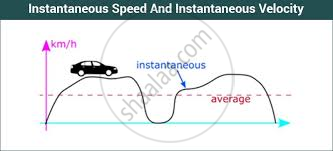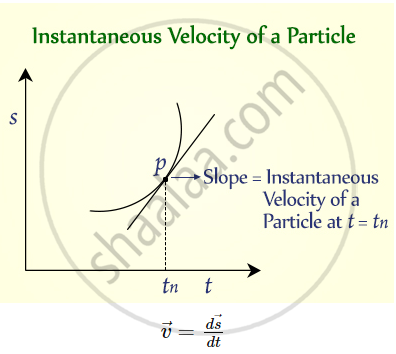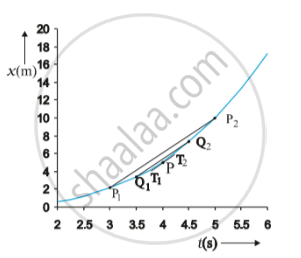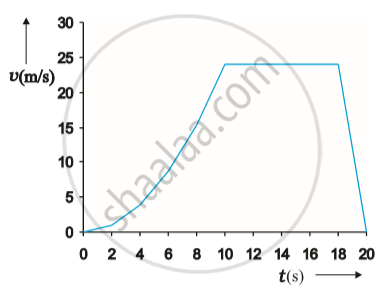# Instantaneous Velocity and Speed

• Speed and Velocity

## Notes

#### Instantaneous velocity and Instantaneous SpeedInstantaneous velocity
The rate of change of displacement of an object in a particular direction is its velocity. Its S.I unit is meter per second.The direction of instantaneous velocity at any time gives the direction of motion of a particle at that point in time. The magnitude of instantaneous velocity equals the instantaneous speed. This happens because, for an infinitesimally small time interval, the motion of a particle can be approximated to be uniform.
v=lim_(Δt→0) (Δx)/(Δt)

We can use the above equation for obtaining the value of velocity at an instant either graphically or numerically. Suppose that we want to obtain graphically the value of velocity at time t = 4 s (point P) for the motion of the car.• The figure has been redrawn in the above Fig. choosing different scales to facilitate the calculation. Let us take ∆t = 2 s centred at t = 4 s.

• Then, by the definition of the average velocity, the slope of line P1P2 (Fig. 3.6) gives the value of average velocity over the interval 3 s to 5 s. Now, we decrease the value of ∆t from 2 s to 1 s. Then line P1P2 becomes Q1Q2 and its slope gives the value of the average velocity over the interval 3.5 s to 4.5 s.

• In the limit ∆t → 0, the line P1P2 becomes tangent to the position time curve at the point P and the velocity at t = 4 s is given by the slope of the tangent at that point. It is difficult to show this process graphically. But if we use numerical method to obtain the value of the velocity, the meaning of the limiting process becomes clear.

It is difficult to show this process graphically. But if we use numerical method to obtain the value of the velocity, the meaning of the limiting process becomes clear.

For the graph shown above, x = 0.08 t^3. Table given below gives the value of ∆x/∆t calculated for ∆t equal to 2.0 s, 1.0 s, 0.5 s, 0.1 s and 0.01 s centred at t = 4.0 s. The second and third columns give the value of

(t-(∆t)/2) and t_2=(t+(∆t)/2)

and the fourth and the fifth columns give the corresponding values of x, i.e. x (t1) = 0.08 t1 3 and x (t2) = 0.08 t2 3. The sixth column lists the difference ∆x = x (t2) – x (t1) and the last column gives the ratio of ∆x and ∆t, i.e. the average velocity corresponding to the value of ∆t listed in the first column. We see from Table that as we decrease the value of ∆t from 2.0 s to 0.010 s, the value of the average velocity approaches the limiting value 3.84 m s–1 which is the value of velocity at
t = 4.0 s, i.e. the value of (dx)/dt at t = 4.0s.

In this manner, we can calculate velocity at each instant for motion of the car. For this case, the variation of velocity with time is found to be as shown in Fig. below.∆t(s) t_1(s) t_2(s) x(t_1)(m) x(t_2)(m) ∆x(m) (∆x)/(∆t)(ms^-1) 2.0 3.0 5.0 2.16 10.0 7.84 3.92 1.0 3.5 4.5 3.43 7.29 3.86 3.86 0.5 3.75 4.25 4.21875 6.14125 1.9225 3.845 0.1 3.95 4.05 4.93039 5.31441 0.38402 3.8402 0.01 3.995 4.005 5.100824 5.139224 0.0384 3.8400

Instantaneous Speed

• The average velocity tells us how fast an object has been moving over a given time interval but does not tell us how fast it moves at different instants of time during that interval. For this, we define instantaneous speed. It is the rate of change of distance with respect to time.
v = (ds)/dt

• Instantaneous speed is always greater than or equal to zero and is a scalar quantity. For uniform motion, instantaneous speed is constant. To understand it in simple words we can say that instantaneous speed at any given time is the magnitude of instantaneous velocity at that time. It is a limit of the average speed as the time interval become very small.

• A moving object does not have the same speed during its travel. Sometimes it speeds up and sometimes slows down. At a given instant time what we read from the speedometer is instantaneous speed. When a cop pulls you over for speeding, he clocked your car’s instantaneous speed or speed at a specific point in time as your car sped down the road.

If you would like to contribute notes or other learning material, please submit them using the button below.

### Shaalaa.com

Straight line motion Part 3 (Speed and velocity) [00:06:22]
S
0%